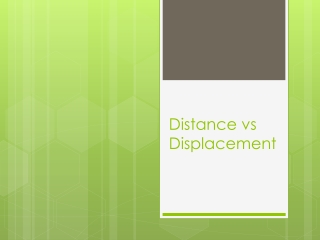DownloadDownload PresentationDistance vs Displacement

# Distance vs Displacement

Download Presentation## Distance vs Displacement

- - - - - - - - - - - - - - - - - - - - - - - - - - - E N D - - - - - - - - - - - - - - - - - - - - - - - - - - -
##### Presentation Transcript

1. Distance vs Displacement

2. Types of Quantities Vector – Quantities that includes both magnitude (size) and a direction • Vectors can be drawn and are represented by arrows Example: 12 m south Scalar – Quantities that do not include direction (magnitude only) Example: 4 seconds

3. Distance vs Displacement • Distance is a scalar quantity: direction doesn’t matter, just how far an object travels • Displacement is a vector • Direction matters • Straight line route from where you started to where you end (as the crow flies) • MUST HAVE DEGREE and DIRECTION

4. What is the Distance traveled? 2 m 1 m 6 m 8 m d = 8 m + 6 m + 2 m + 1 m d = 17 m

5. What is the displacement? 2 m 1 m 6 m 8 m

6. Distance vs. Displacement Examples 6 m 3 m Distance= Displacement = 9 m 9 m right 3 m 6 m Distance= Displacement = 9 m 3 m left Distance= Displacement = 9 m ? 3 m 6 m

7. Distance versus Displacement • To determine displacement draw a diagram of the path taken • Example: 8 m east, then 6m north, then 2 m east, then 1 m south • Simplify the diagram to a right triangle by adding east arrows and north arrows. • Use Pythagorean Theorem to determine the displacement 2 m 1 m 6 m 8 m 1 m 6 m 5 m 8 m 10 m 2 m

8. Range of Values • Maximum Displacement occurs when: • The two vectors are pointed in the same direction (0o) • Example: • Displacement = 10 m • Minimum Displacement occurs when: • The two vectors are pointed in the opposite directions (180o) • Example: • Displacement= 6 m Right • All other angles will result in a value in between that range. 8 m 2 m 2 m 8 m

9. Example with Word Problems • A turtle crawls 5m north, then 3m east, and then 2 m south in 1000 seconds. What is his distance traveled, displacement, average speed and average velocity? • Givens: t=1000 s 3 m 2 m 5 m

10. Distance vs Displacement • Distance= • 5m +3m +2m = 10 m • Displacement= • a2 + b2 = c2 • (3m)2 +(3m)2 = c2 • 18 m2 = c2 • c = 4.2 m 37o Northeast 3 m 3 m